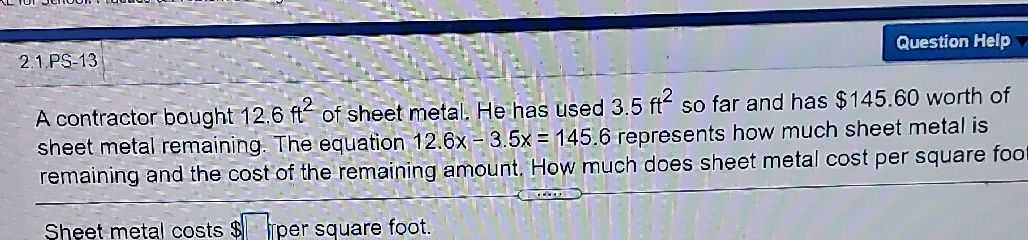### ¿Todavía tienes preguntas de matemáticas?

Pregunte a nuestros tutores expertos
Algebra
PreguntaA contractor bought $$12.6 ft ^ { 2 }$$ of sheet metal. He has used $$3.5 ft ^ { 2 }$$ so far and has $$\ 145.60$$ worth of sheet metal remaining. The equation $$12.6 x - 3.5 x = 145.6$$ represents how much sheet metal is remaining and the cost of the remaining amount. How much does sheet metal cost per square foo Sheet metal costs     per square foot.